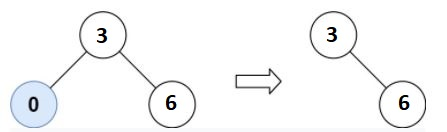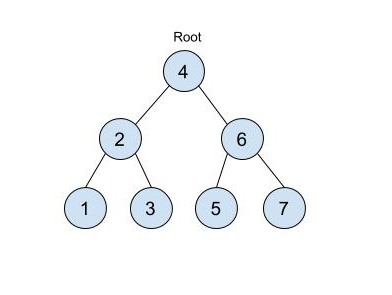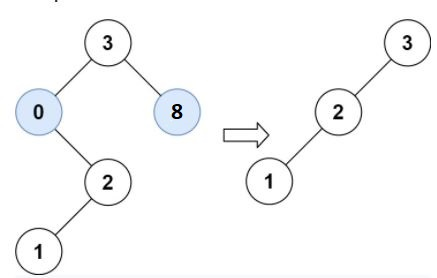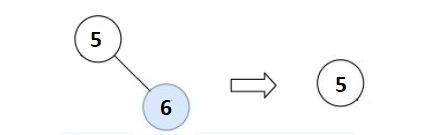3

# Modify BST

Difficulty: MEDIUMContributed By
Shrey Pansuria
Avg. time to solve
20 min
Success Rate
80%

Problem Statement

#### For example: For ‘LOW’ = 3 and ‘HIGH’ = 6 see the below picture for reference:``````As we can see 0 is not in the range [3, 6] so we delete this node from the ‘TREE’.
``````
##### Input Format:
``````The first line of input contains an integer 'T' representing the number of test cases. Then the test cases follow.

The first line of each test case contains two single space-separated integers ‘LOW’ and ‘HIGH’.

The second line of each test case contains elements in the level order form. The line consists of values of nodes separated by a single space. In case a node is null, we take -1 in its place.

For example, the input for the tree depicted in the below image would be :
````````````4
2 6
1 3 5 7
-1 -1 -1 -1 -1 -1
``````

#### Explanation :

``````Level 1 :
The root node of the tree is 4

Level 2 :
Left child of 4 = 2
Right child of 4 = 6

Level 3 :
Left child of 2 = 1
Right child of 2 = 3
Left child of 6 = 5
Right child of 6 = 7

Level 4 :
Left child of 1 = null (-1)
Right child of 1 = null (-1)
Left child of 3 = null (-1)
Right child of 3 = null (-1)
Left child of 5 = null (-1)
Right child of 5 = null (-1)
Left child of 7 = null (-1)
Right child of 7 = null (-1)

The first not-null node(of the previous level) is treated as the parent of the first two nodes of the current level. The second not-null node (of the previous level) is treated as the parent node for the next two nodes of the current level and so on.
The input ends when all nodes at the last level are null(-1).
``````
##### Note :
``````The above format was just to provide clarity on how the input is formed for a given tree.

The sequence will be put together in a single line separated by a single space. Hence, for the above-depicted tree, the input will be given as:

4 2 6 1 3 5 7 -1 -1 -1 -1 -1 -1
``````
##### Output Format :
``````For each test case, print the modified tree after trimming in the level order form.

The output for each test case is printed in a separate line.
``````
##### Note:
``````You do not need to print anything, it has already been taken care of. Just implement the given function.
``````
##### Constraints :
``````1 <= T <= 100
1 <= N <= 10 ^ 4
1 <= DATA <= 10 ^ 4
1 <= LOW , HIGH <= 10 ^ 4

Where N is the number of nodes in the ‘TREE’, ‘DATA’ represents the value of the node.

Time Limit: 1 Sec
``````
##### Sample Input 1 :
``````2
1 5
3 -1 -1
2 8
1 0 9 -1 -1 -1 -1
``````
##### Sample Output 1:
``````3 -1 -1
-1
``````

#### Explanation Of Sample Output 1:

``````For the first test case :
There is only one node in the ‘TREE’ which lies in the given range 1 <= 3 <= 5
So in this case we don’t need to delete any node from the ‘TREE’.

For the second test case :
All the nodes in the ‘TREE’ are not in the range so every node gets deleted from the ‘TREE’. So we return NULL in this case.
``````
##### Sample Input 2 :
``````2
1 6
3 0 8 -1 2 -1 -1 1 -1 -1 -1
5 5
5 -1 6 -1 -1
``````
##### Sample Output 2 :
``````3 2 -1 1 -1 -1 -1
5 -1 -1
``````

#### Explanation Of Sample Output 2:

``````For the first test case :
Nodes marked blue are not in the range so we will delete these nodes.
````````````For the second test case :
Node marked blue is not in the range so we will delete this node.
``````Console